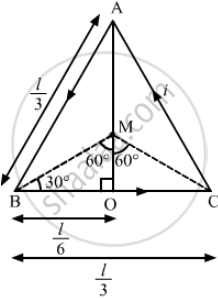Advertisement Remove all ads

# A Wire of Length L Is Bent in the Form of an Equilateral Triangle and Carries an Electric Current I. Find the Magnetic Field B At the Centre. - Physics

Short Note

A wire of length l is bent in the form of an equilateral triangle and carries an electric current i. Find the magnetic field B at the centre.

Advertisement Remove all ads

#### Solution

Let ABC be the equilateral triangle with side l/3 and centre M.$\text{ In } ∆ AOB,$
$AO = \sqrt{\left( \frac{l}{3} \right)^2 - \left( \frac{I}{6} \right)^2}$
$= l\sqrt{\frac{1}{9} - \frac{1}{36}} = l\sqrt{\frac{4 - 1}{36}} = l\sqrt{\frac{1}{12}}$
$\therefore \text{ MO }= \frac{1}{3} \times l\sqrt{\frac{1}{12}} = \frac{l}{6\sqrt{3}}$

The angles made by points B and C with centre M are  $\theta_1 = 60^\circ \text{ and } \theta_2 = 60^\circ$

Separation of the point from the wire, d = MO = $\frac{l}{6\sqrt{3}}$
Thus, the magnetic field due to current in wire BC is given by
$B = \frac{\mu_0 i}{4\pi d}(\sin \theta_1 + \sin \theta_2 )$
$\Rightarrow B = \frac{\mu_0 i}{4\pi\frac{l}{6\sqrt{3}}}(\sin 60 + \sin 60)$
$\Rightarrow B = \frac{\mu_0 i}{4\pi l}6\sqrt{3} \times \sqrt{3}$
Now,
Net magnetic field at M = Magnetic field due to wire BC + Magnetic field due to wire CA + Magnetic field due to wire AB
Since all wires are the same  $B_{net} = 3B$

$= \frac{27 \mu_0 i}{\pi l}$
It is perpendicular to the plane in outward direction if the current is anticlockwise and perpendicular to the plane in inward direction if the current is clockwise.

Is there an error in this question or solution?
Advertisement Remove all ads

#### Video TutorialsVIEW ALL 

Advertisement Remove all ads
Share
Notifications

View all notifications

Forgot password?